# Quantum supremacy? Not so fast.

By
Jonas Araujo
December 21, 2021
7 minutes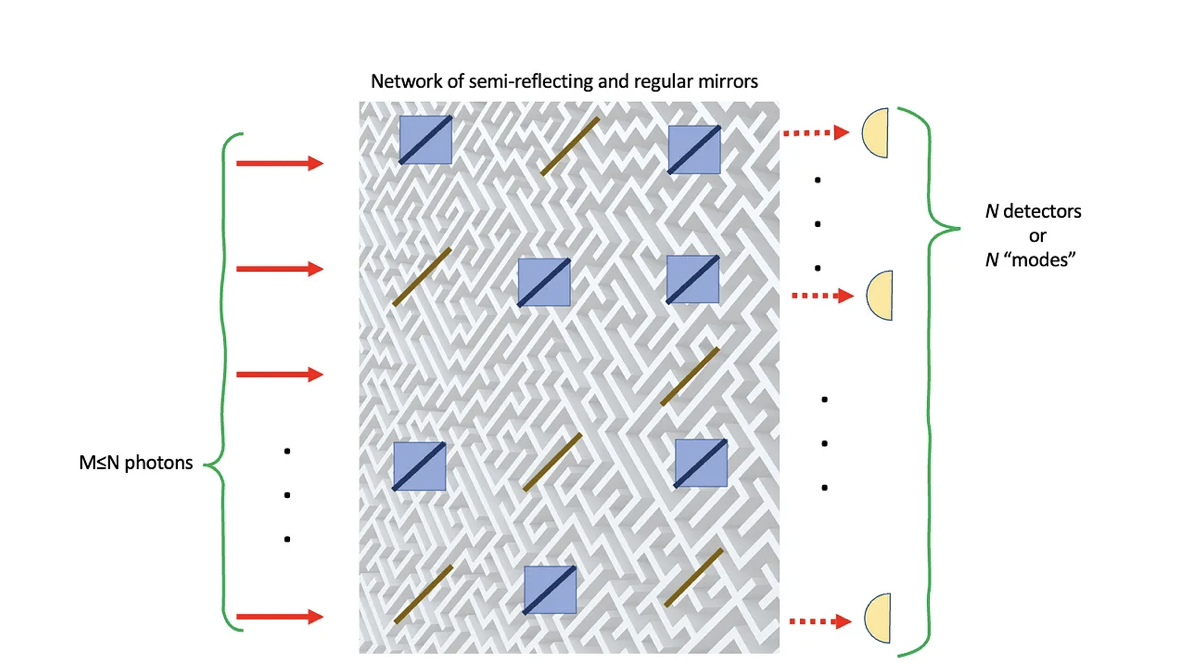When Google claimed quantum supremacy with its 53-qubit quantum processing unit , IBM challenged it claiming that “[quantum supremacy] has not been met” .

They argued that a classical computer could solve the problem in 2.5 days with far more accuracy, debunking Google’s claims on grounds of John Preskill’s definition of quantum supremacy: “[it] describes the point where quantum computers can do things that classical computers can’t” . The problem at hand was a random number generator via a large quantum circuit.

More recently, the Chinese computers, Zuchongzi and Jiuzhang 2.0 , have also claimed quantum supremacy in a (similar) task called boson sampling. But what problem is this and why does it pose a computational challenge? Is it a useful problem?

## Boson Sampling

If you look it up on Wikipedia , two statements might stand out. Here they are:

“[boson sampling] consists of sampling from the probability distribution of identical bosons scattered by a linear interferometer”

Boson sampling constitutes a restricted model of non-universal quantum computation”

In the first there are some technical terms, let’s go over them, starting by bosons.

All fundamental particles in nature can be categorized either as bosons or fermions. This classification loosely describes how social they are if they are indistinguishable. For example, suppose we have a tiny well where quantum particles can be confined. Schrödinger equation tell us that only particles with certain energies are allowed into the well.

If we’re talking about identical bosons, they are quite social and can share the same energy level.

Identical fermions, on the hand, are anti-social and will not share the same energy level, much like people who will leave a party if someone is wearing the same t-shirt or dress.

Natural candidates for boson sampling are photons, particles of light. The light, formed by a collection of photons is scattered by a linear interferometer. An example of it is the Mach-Zehnder interferometer.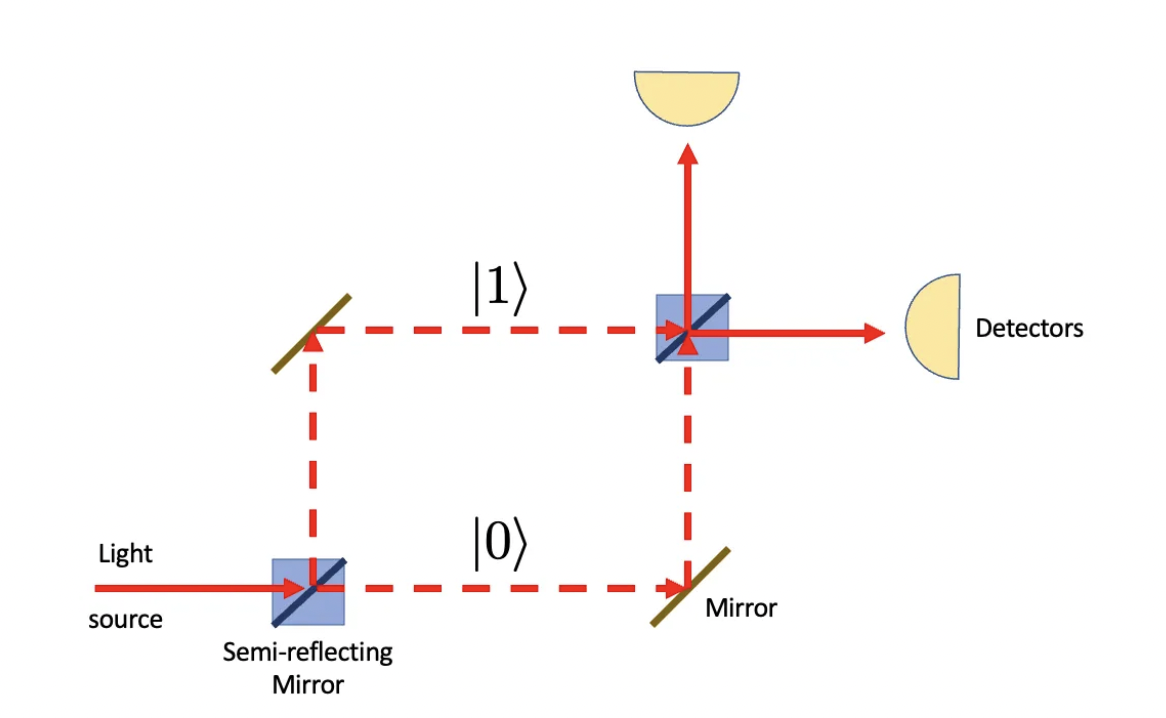A simple example of Mach-Zehnder interferometer, like ones used in the quantum eraser experiment.

The light is split in a semi-reflecting mirror and then recombined to be detected. The quantum superpositions appear as each photon passes through both paths simultaneously. Accordingly, larger linear interferometers are just a collection of mirrors and semi-reflecting mirrors that split and recombine light.

Recapitulating, we have a set of semi-reflecting and regular mirrors, identical photons, and single-photon detectors.

Wrapping up the first statement, probability sampling is nothing but a collection of results from individual detections for each photon that goes through the interferometer. But why is it so difficult to emulate it in a classical computer? Let’s give it a deeper look!

Here is a typical structure of a linear interferometer:The linear interferometer corresponds to a network or a maze of semi-reflecting and regular mirrors.

It can have N modes (that means N possible entries and as many detectors in the end), that should be fed with M (at most equal to N) identical photons. Each incoming photon can be reflected or go through each semi-reflecting mirror until it gets to one of the detectors. Also, have in mind these circuit elements do not entangle photons. Now to the mathematics of it!

Each time a photon interacts or emerges from a process, we say it was destroyed or created, respectively. These actions of creation and destruction (or annihilation) are done by operators.

Do not be intimidated, this just means the detection of a photon in detector depends on the following creation operator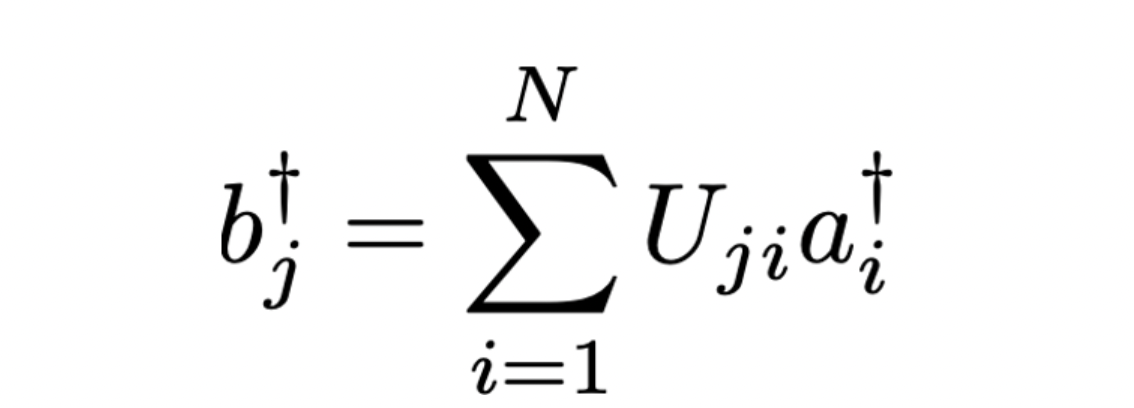The operator a-dagger creates a photon that enters in the interferometer, while b-dagger creates a photon that will be counted by the detectors.

“The creation and detection of a photon in detector depends on all the inputs of the interferometer and on how the semi-reflecting and regular mirrors are organized in the interferometer”

The unitary operator U ensures the number of photons does not change and contains all the information regarding the probability distribution in the detectors. The question is, how to obtain the probability distributions from the operator U?

Let’s illustrate how to do it with the following problem:

Suppose we input M identical photons in the first modes, one in each input slot. What is the probability p(a, b, c, …, n) of measuring a, b, c, …, n photons in detectors 1, 2, 3, …, N, respectively?

(of course a, b, c, …, n should add up to M photons)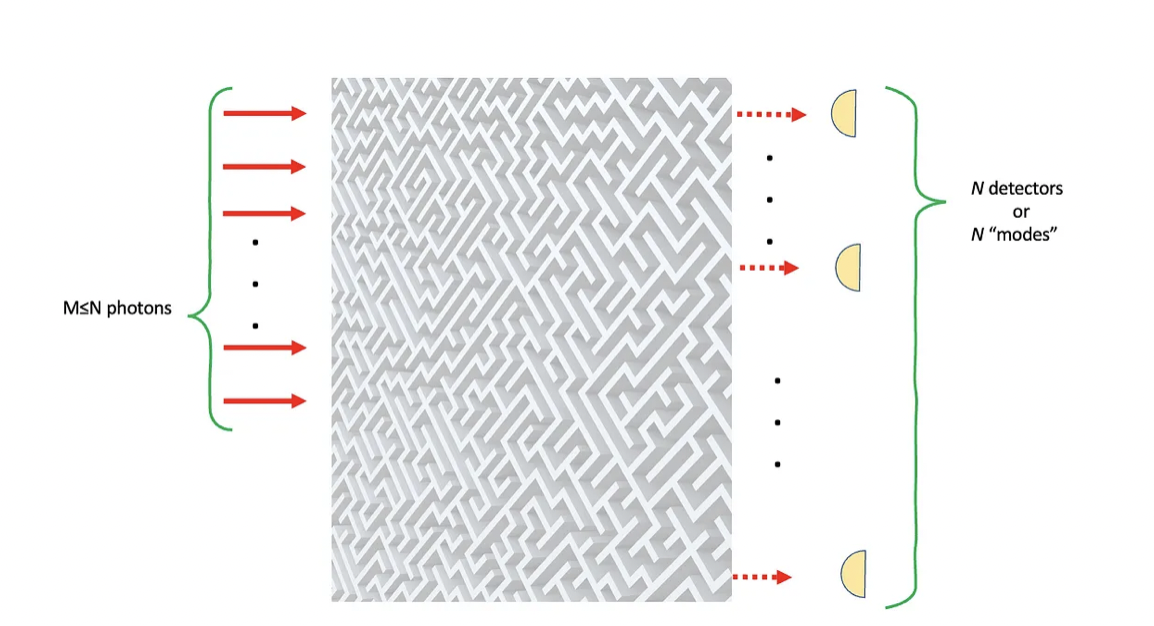M photons enter the interferometer and are scattered by the mirrors to produce a probabilistic distribution.

Quantum mechanics tells us this probability is given by 

where perm stands for the permanent of the matrix U_T, which is found from the matrix U by keeping only its first M columns and by repeating the k-th row by its respective photon count. It is easier to see with an example!

Say we have 5 detectors and input M=4 photons, and we are looking for the probability of measuring two photons in detector 1, one in detector 3 and one in detector 4. We then have:

M=4

N=5

{a, b, c, d, n} = {2, 0, 1, 1, 0}, that means duplicate the first row, keep the third and fourth, and eliminate the second and fifth.

These imply we must do the following on the operator U

Obtaining then

whose permanent must be found, and that is where the difficulty arises.

We rarely hear about the permanent of a matrix, despite its similarity with its much more popular sibling, the determinant. While the determinant of a matrix has terms that pick up negative signs for different permutations, the terms for the permanent are always added. For 2x2 matrices, we have:

while the determinant of it would be just ad — bc.

It turns out this relative sign makes the evaluation of permanents fall into the #P-hard complexity class, and there are no efficient classical algorithms to solve it, nor even to find it up to a multiplicative factor . In fact, there would be groundbreaking consequences if such an algorithm were found.

But why does boson sampling demand permanents? The answer is in its interpretation!

Permanents count permutations, much like determinants count areas, and the boson sampling problem is fundamentally a (probabilistic) permutation problem of identical elements. On top of that, these identical elements can occupy the same quantum state, adding complexity to it.

However difficult, boson sampling is not useful for other problems. It does not calculate sums, for example.

This has to do with the second statement, the one about the non-universality of boson sampling. Well, the explanation for this is much simpler!

A universal quantum computer should have at least: single-qubit rotation gates, a phase-shift gate and a CNOT gate. The boson sampling problem, however, has no entanglement operators (the mirrors do not entangle photons), so a CNOT gate cannot be built. In other words: no entanglement, no universal computation! That is why boson sampling is limited and is not generally useful outside its scope. That said, photon-based universal quantum computers must have nonlinear (entangling) elements in photonic circuits, and there is a recent proposal for it .

Fortunately, the current computers based on superconducting qubits are already universal. These noisy intermediate scale quantum (NISQ) computers are nevertheless subject to errors, meaning they cannot sustain long calculations. This is why the most promising approaches invoke hybrid set-ups of classical and quantum computers to draw the best of both worlds.

The idea is bringing quantum advantage instead of quantum supremacy. It stands for any advantage quantum computing offers over classical computing, such as lower runtimes or energy consumption.

At ColibrITD our goal is bringing Quantum Computing for All. We use the already available universal quantum computers to obtain the most quantum advantage for our clients’ use cases.

References

 [https://www.ibm.com/blogs/research/2019/10/on-quantum-supremacy/]

 [https://quantum-journal.org/papers/q-2018-08-06-79/]

 [https://spectrum.ieee.org/quantum-computing-china]

 [https://arxiv.org/abs/quant-ph/0406127]

 [https://en.wikipedia.org/wiki/♯P-completeness_of_01-permanent]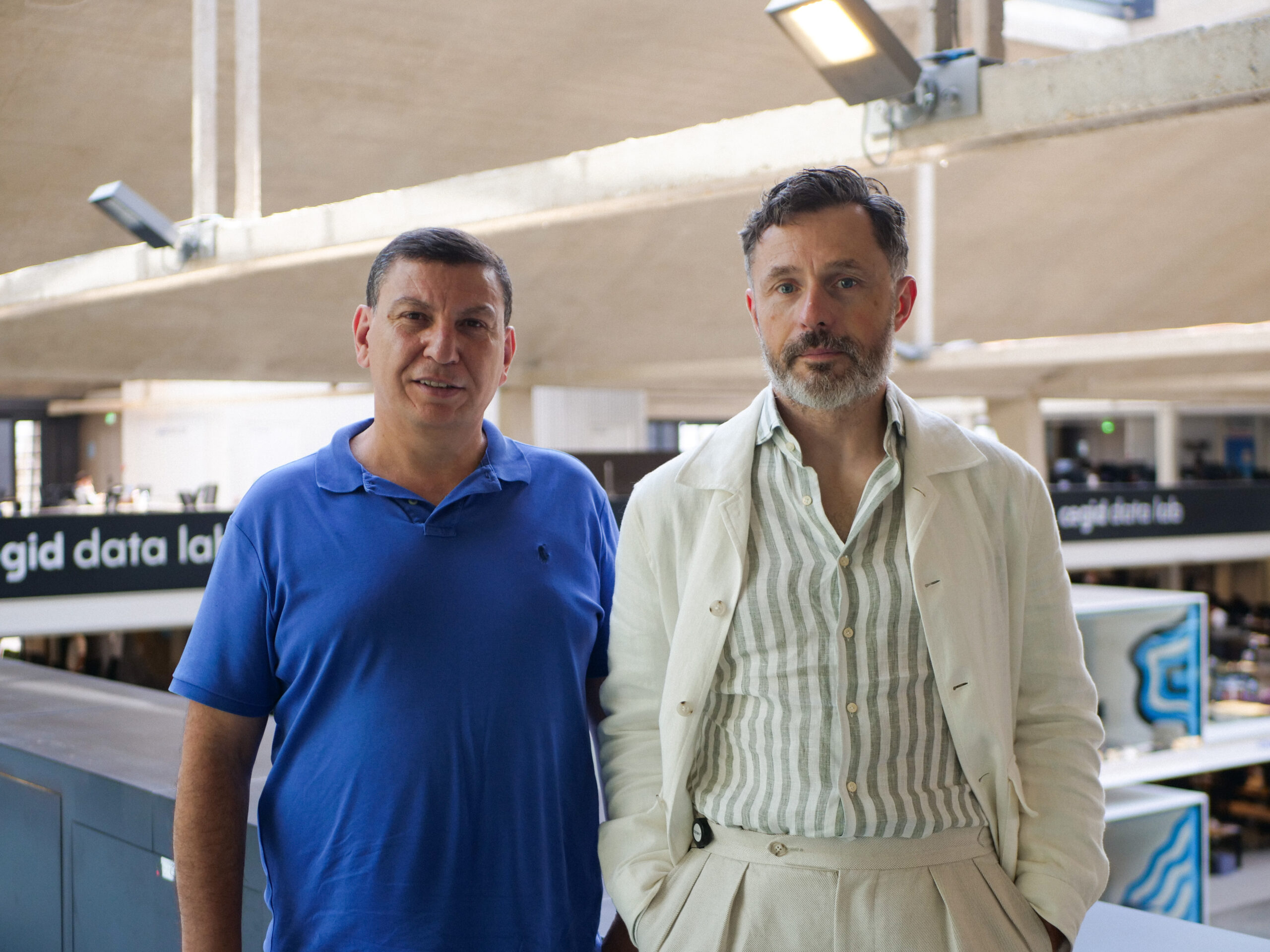Jonas Araujo